# Michigan Merit Exam - Math: Triangles & Trigonometry Chapter Exam

Exam Instructions:

Choose your answers to the questions and click 'Next' to see the next set of questions. You can skip questions if you would like and come back to them later with the yellow "Go To First Skipped Question" button. When you have completed the practice exam, a green submit button will appear. Click it to see your results. Good luck!

### Page 1

#### Question 1 1. In the pictured triangle, side b is 12 and side c is 16. If ∠A is 84 degrees, then approximately how long is side a? Round the value of the cosine to the nearest whole number.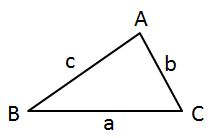#### Question 2 2. In the pictured triangle, ∠A is 98 degrees and ∠B is 12 degrees. If side a is 84 units long, approximately how long is side b?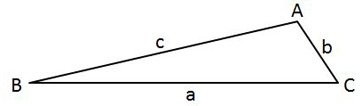#### Question 4 4. In the pictured triangle, ∠A is 58 degrees and ∠C is 63 degrees. If side a is 84 units long, approximately how long is side c?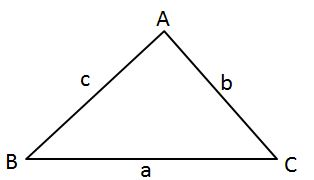#### Question 5 5. In the pictured triangle, side a is 9 and side b is 6. If ∠C is 26 degrees, then approximately how long is side c? Round the value of the cosine to the nearest whole number.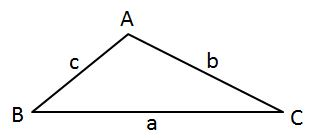### Page 2

#### Question 6 6. In the pictured triangle, side a is 32, side b is 24, and side c is 37. What is the approximate measure of ∠C? When calculating the inverse cosine, round the initial value to the nearest whole number.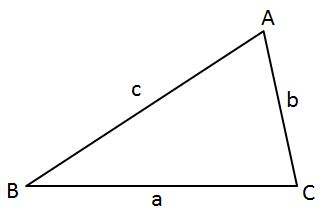#### Question 7 7. In the pictured triangle, side a is 23, side b is 18, and side c is 16. What is the approximate measure of ∠C? When calculating the inverse cosine, round the initial value to the nearest whole number.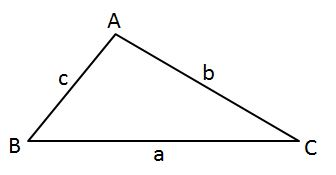#### Question 8 8. In the pictured triangle, ∠A is 81 degrees and ∠B is 67 degrees. If side a is 34 units long, approximately how long is side b?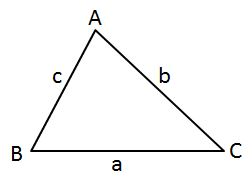#### Question 10 10. In the pictured triangle, side a is 17 and side c is 20. If ∠B is 19 degrees, then approximately how long is side b? Round the value of the cosine to the nearest whole number.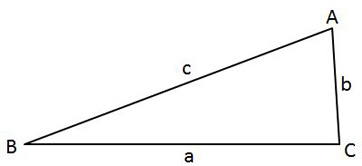### Page 3

#### Question 13 13. What is x?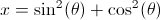#### Question 15 15. In the pictured triangle, ∠A is 137 degrees and ∠B is 28 degrees. If side b is 71 units long, approximately how long is side a?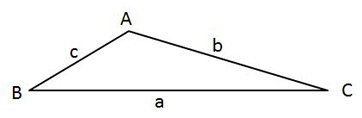### Page 4

#### Question 16 16. In the pictured triangle, ∠A is 62 degrees and ∠C is 86 degrees. If side a is 112 units long, approximately how long is side c?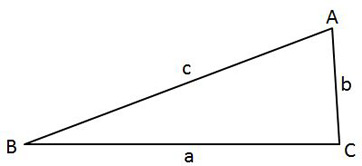#### Question 17 17. What is z in terms of x and y?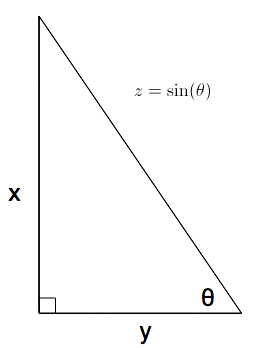#### Question 18 18. What is x?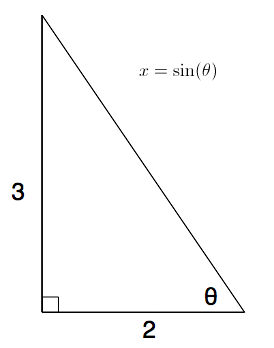#### Question 19 19. What is z in terms of x?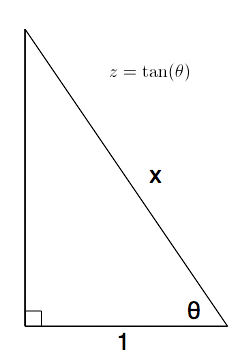#### Michigan Merit Exam - Math: Triangles & Trigonometry Chapter Exam Instructions

Choose your answers to the questions and click 'Next' to see the next set of questions. You can skip questions if you would like and come back to them later with the yellow "Go To First Skipped Question" button. When you have completed the practice exam, a green submit button will appear. Click it to see your results. Good luck!

Support